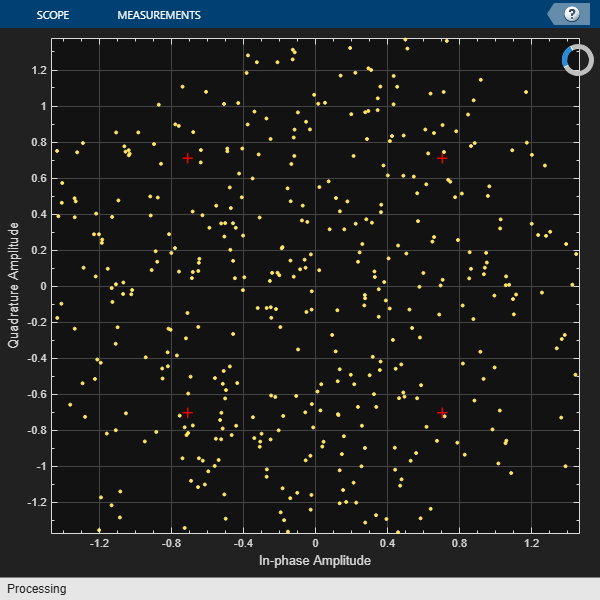This example shows how to use Rayleigh and Rician multipath fading channel System objects and their built-in visualization to model a fading channel. Rayleigh and Rician fading channels are useful models of real-world phenomena in wireless communication. These phenomena include multipath scattering effects, time dispersion, and Doppler shifts that arise from relative motion between the transmitter and receiver.

Processing a signal using a fading channel involves the following steps:

1. Create a channel System object™ that describes the channel that you want to use. A channel object is a type of MATLAB® variable that contains information about the channel, such as the maximum Doppler shift.

2. Adjust properties of the System object, if necessary, to tailor it to your needs. For example, you can change the path delays or average path gains.

3. Apply the channel System object to your signal using the step method, which generates random discrete path gains and filters the input signal.

The characteristics of a channel can be shown with the built-in visualization support of the System object.

### Initialization

The following variables control both the Rayleigh and Rician channel objects. By default, the channel is modeled as four fading paths, each representing a cluster of multipath components received at around the same delay.

```sampleRate500kHz = 500e3; % Sample rate of 500K Hz sampleRate20kHz = 20e3; % Sample rate of 20K Hz maxDopplerShift = 200; % Maximum Doppler shift of diffuse components (Hz) delayVector = (0:5:15)*1e-6; % Discrete delays of four-path channel (s) gainVector = [0 -3 -6 -9]; % Average path gains (dB) ```

The maximum Doppler shift is computed as v*f/c, where v is the mobile speed, f is the carrier frequency, and c is the speed of light. For example, a maximum Doppler shift of 200 Hz (as above) corresponds to a mobile speed of 65 mph (30 m/s) and a carrier frequency of 2 GHz.

By convention, the delay of the first path is typically set to zero. For subsequent paths, a 1 microsecond delay corresponds to a 300 m difference in path length. In some outdoor multipath environments, reflected paths can be up to several kilometers longer than the shortest path. With the path delays specified above, the last path is 4.5 km longer than the shortest path, and thus arrives 15 microseconds later.

Together, the path delays and path gains specify the channel's average delay profile. Typically, the average path gains decay exponentially with delay (i.e., the dB values decay linearly), but the specific delay profile depends on the propagation environment. In the delay profile specified above, we assume a 3 dB decrease in average power for every 5 microseconds of path delay.

The following variables control the Rician channel System object. The Doppler shift of the specular component is typically smaller than the maximum Doppler shift (above) and depends on the mobile's direction of travel relative to the direction of the specular component. The K-factor specifies the linear ratio of average received power from the specular component relative to that of the associated diffuse components.

```KFactor = 10; % Linear ratio of specular power to diffuse power specDopplerShift = 100; % Doppler shift of specular component (Hz) ```

### Creating Channel System Objects

With the parameters specified above, we can now create the `comm.RayleighChannel` and `comm.RicianChannel` System objects. We configure the objects to use their self-contained random stream with a specified seed for path gain generation.

```% Configure a Rayleigh channel object rayChan = comm.RayleighChannel( ... 'SampleRate', sampleRate500kHz, ... 'PathDelays', delayVector, ... 'AveragePathGains', gainVector, ... 'MaximumDopplerShift', maxDopplerShift, ... 'RandomStream', 'mt19937ar with seed', ... 'Seed', 10, ... 'PathGainsOutputPort', true); % Configure a Rician channel object ricChan = comm.RicianChannel( ... 'SampleRate', sampleRate500kHz, ... 'PathDelays', delayVector, ... 'AveragePathGains', gainVector, ... 'KFactor', KFactor, ... 'DirectPathDopplerShift', specDopplerShift, ... 'MaximumDopplerShift', maxDopplerShift, ... 'RandomStream', 'mt19937ar with seed', ... 'Seed', 100, ... 'PathGainsOutputPort', true); ```

### Modulation and Channel Filtering

Create a `comm.QPSKModulator` System object to modulate the channel data, which has been generated using the RANDI function. Note that in the code below a 'frame' refers to a vector of information bits. A phase offset of pi/4 is used for this example.

```qpskMod = comm.QPSKModulator( ... 'BitInput', true, ... 'PhaseOffset', pi/4); % Number of bits transmitted per frame is set to be 1000. For QPSK % modulation, this corresponds to 500 symbols per frame. bitsPerFrame = 1000; msg = randi([0 1],bitsPerFrame,1); % Modulate data for transmission over channel modSignal = qpskMod(msg); % Apply Rayleigh or Rician channel object on the modulated data rayChan(modSignal); ricChan(modSignal); ```

### Visualization

The fading channel System objects have built-in visualization to show the channel impulse response, frequency response, or Doppler spectrum from the `step` method. To invoke it, set the `Visualization` property to its desired value before calling the `step` method. Release the Rayleigh and Rician channel System objects now so that we can change their property values.

```release(rayChan); release(ricChan); ```

Setting the `Visualization` property to `'Impulse response'` shows the bandlimited impulse response (yellow circles). The visualization also shows the delays and magnitudes of the underlying fading path gains (pink stembars) clustered around the peak of the impulse response. Note that the path gains do not equal the `AveragePathGains` property value because the Doppler effect causes the gains to fluctuate over time.

Similarly, setting the `Visualization` property to `'Frequency response'` shows the frequency response (DFT transformation) of the impulses. You can also set `Visualization` to `'Impulse and frequency responses'` to display both impulse and frequency responses side by side. .

You can control the percentage of the input samples to be visualized by changing the `SamplesToDisplay` property. In general, the smaller the percentage, the faster the simulation runs. Once the visualization figure opens up, click the `Playback` button and turn off the "Reduce Updates to Improve Performance" or "Reduce Plot Rate to Improve Performance" option to further improve display accuracy. The option is on by default for faster simulation. To see the channel response for every input sample, uncheck this option and set `SamplesToDisplay` to `'100%'`.

```rayChan.Visualization = 'Impulse and frequency responses'; rayChan.SamplesToDisplay = '100%'; numFrames = 2; for i = 1:numFrames % Display impulse and frequency responses for 2 frames % Create random data msg = randi([0 1],bitsPerFrame,1); % Modulate data modSignal = qpskMod(msg); % Filter data through channel and show channel responses rayChan(modSignal); end ```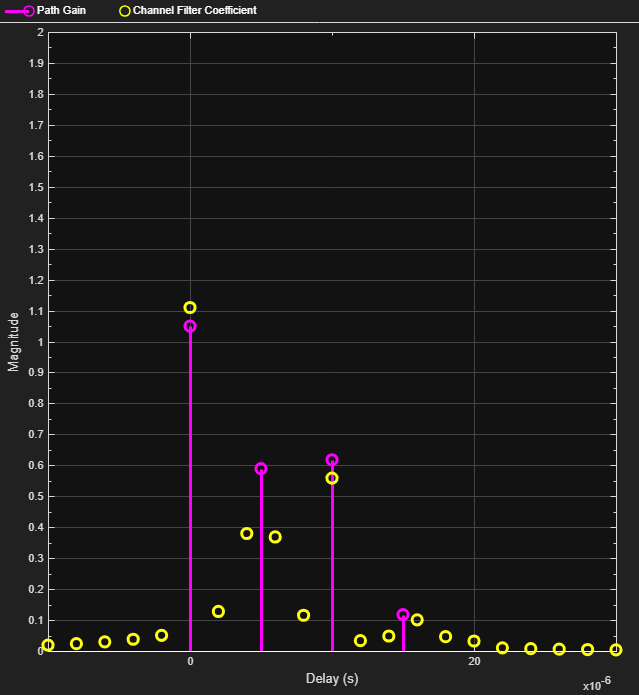As you can see, the channel frequency response is not flat and may have deep fades over the 500K Hz bandwidth. Because the power level varies over the signal's bandwidth, it is referred to as frequency-selective fading.

For the same channel specification, we now display the Doppler spectrum for its first discrete path, which is a statistical characterization of the fading process. The System object makes periodic measurements of the Doppler spectrum (blue stars). Over time with more samples processed by the System object, the average of this measurement better approximates the theoretical Doppler spectrum (yellow curve).

```release(rayChan); rayChan.Visualization = 'Doppler spectrum'; numFrames = 5000; for i = 1:numFrames % Display Doppler spectrum from 5000 frame transmission msg = randi([0 1],bitsPerFrame,1); modSignal = qpskMod(msg); rayChan(modSignal); end ```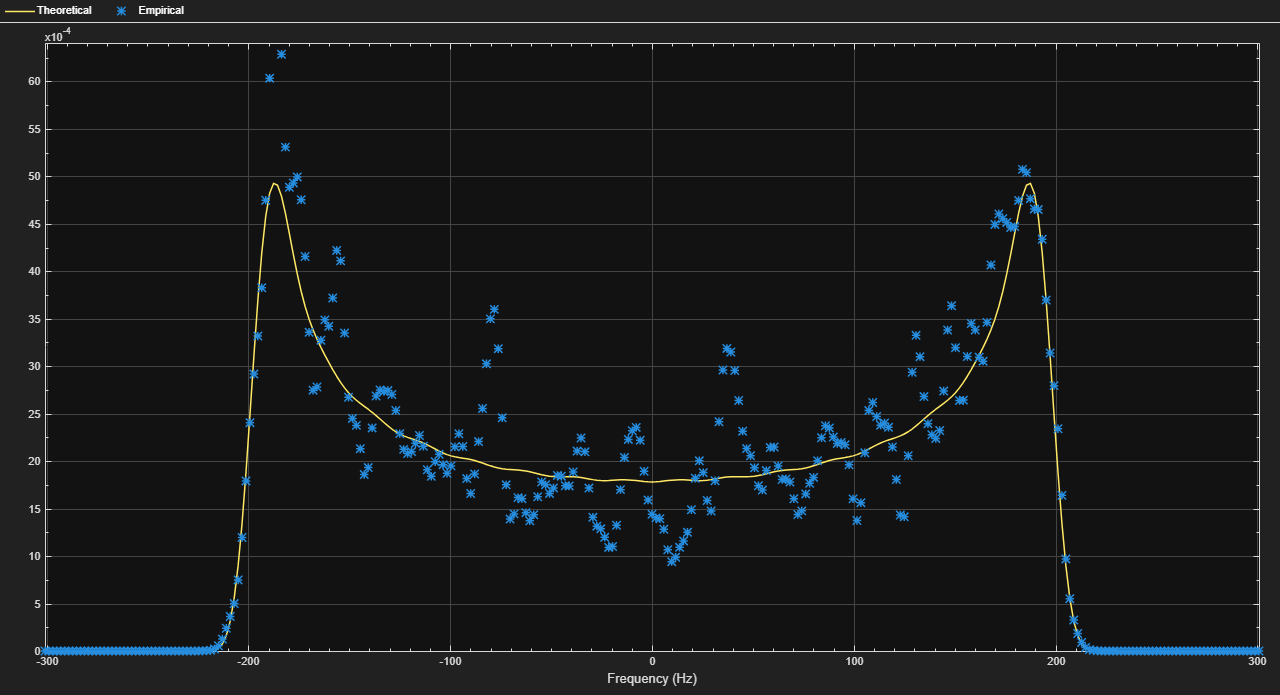When the bandwidth is too small for the signal to resolve the individual components, the frequency response is approximately flat because of the minimal time dispersion caused by the multipath channel. This kind of multipath fading is often referred to as narrowband fading, or frequency-flat fading.

We now reduce the signal bandwidth from 500 kb/s (250 ksym/s) to 20 kb/s (10 ksym/s), so the channel's delay span (15 microseconds) is much smaller than the QPSK symbol period (100 microseconds). The resultant impulse response has very small intersymbol interference (ISI) and the frequency response is approximately flat.

```release(rayChan); rayChan.Visualization = 'Impulse and frequency responses'; rayChan.SampleRate = sampleRate20kHz; rayChan.SamplesToDisplay = '25%'; % Display one of every four samples numFrames = 2; for i = 1:numFrames % Display impulse and frequency responses for 2 frames msg = randi([0 1],bitsPerFrame,1); modSignal = qpskMod(msg); rayChan(modSignal); end ```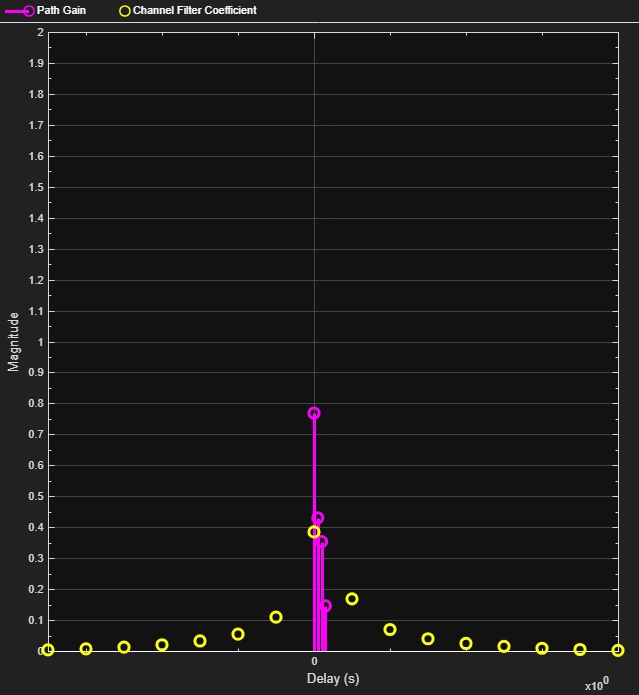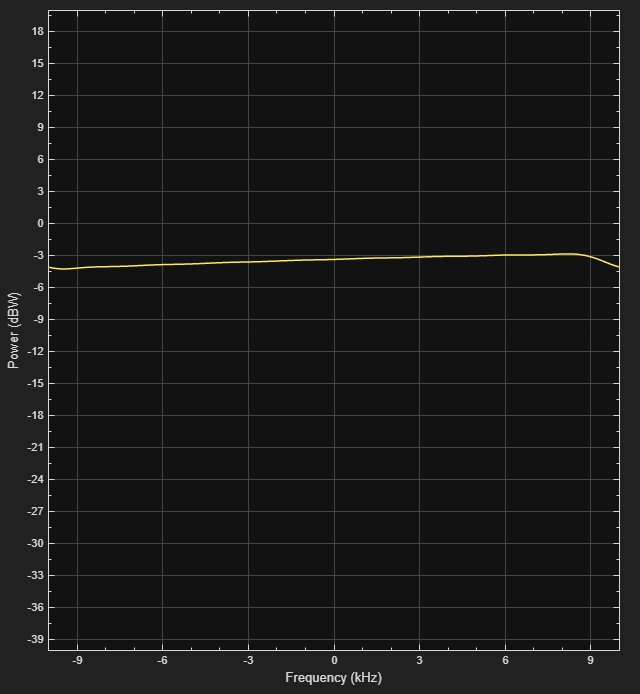To simplify and speed up modeling, narrowband fading channels are typically modeled as a single-path fading channel. That is, a multipath fading model overspecifies a narrowband fading channel. The following settings correspond to a narrowband fading channel. Notice that the shape of the bandlimited impulse response is flat.

```release(rayChan); rayChan.PathDelays = 0; % Single fading path with zero delay rayChan.AveragePathGains = 0; % Average path gain of 1 (0 dB) for i = 1:numFrames % Display impulse and frequency responses for 2 frames msg = randi([0 1],bitsPerFrame,1); modSignal = qpskMod(msg); rayChan(modSignal); end ```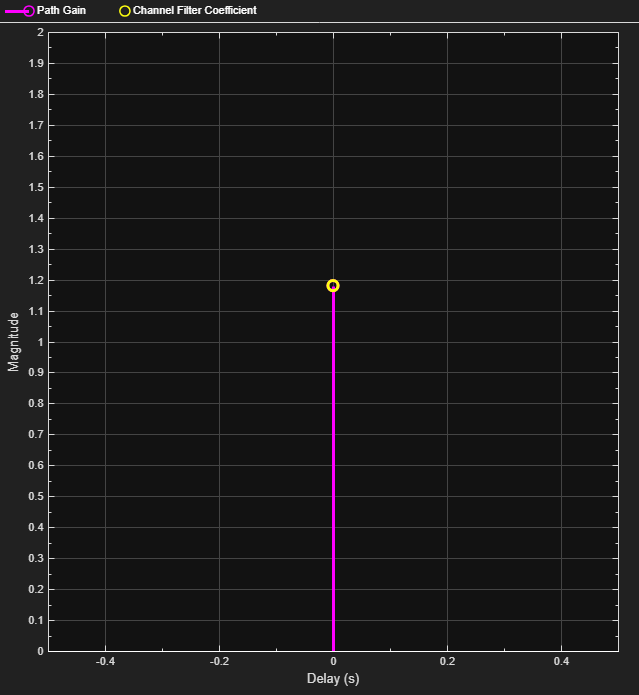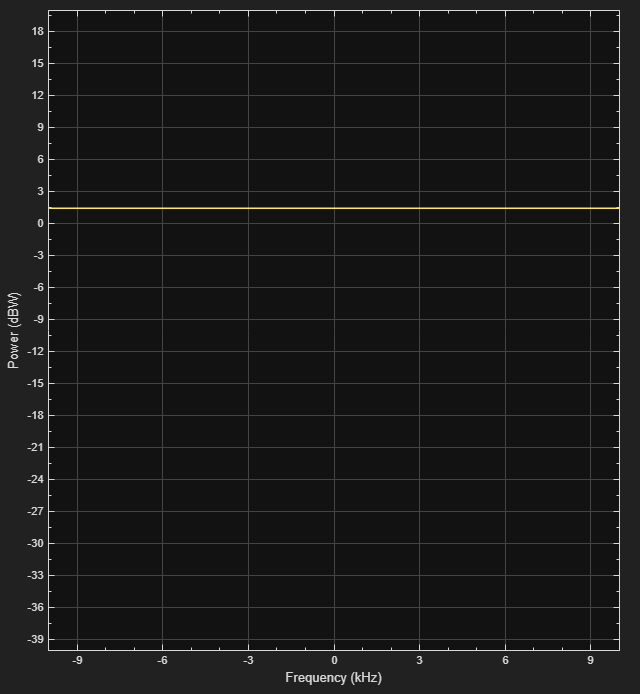The Rician fading channel System object models line-of-sight propagation in addition to diffuse multipath scattering. This results in a smaller variation in the magnitude of path gains. To compare the variation between Rayleigh and Rician channels, we make use of a `dsp.TimeScope` System object to view their path gains over time. Note that the magnitude fluctuates over approximately a 10 dB range for the Rician fading channel (blue curve), compared with 30-40 dB for the Rayleigh fading channel (yellow curve). For the Rician fading channel, this variation would be further reduced by increasing the K-factor (currently set to 10).

```release(rayChan); rayChan.Visualization = 'Off'; % Turn off System object's visualization ricChan.Visualization = 'Off'; % Turn off System object's visualization % Same sample rate and delay profile for the Rayleigh and Rician objects ricChan.SampleRate = rayChan.SampleRate; ricChan.PathDelays = rayChan.PathDelays; ricChan.AveragePathGains = rayChan.AveragePathGains; % Configure a Time Scope System object to show path gain magnitude gainScope = dsp.TimeScope( ... 'SampleRate', rayChan.SampleRate, ... 'TimeSpan', bitsPerFrame/2/rayChan.SampleRate, ... % One frame span 'Name', 'Multipath Gain', ... 'ShowGrid', true, ... 'YLimits', [-40 10], ... 'YLabel', 'Gain (dB)'); % Compare the path gain outputs from both objects for one frame msg = randi([0 1],bitsPerFrame,1); modSignal = qpskMod(msg); [~, rayPathGain] = rayChan(modSignal); [~, ricPathGain] = ricChan(modSignal); % Form the path gains as a two-channel input to the time scope gainScope(10*log10(abs([rayPathGain, ricPathGain]).^2)); ```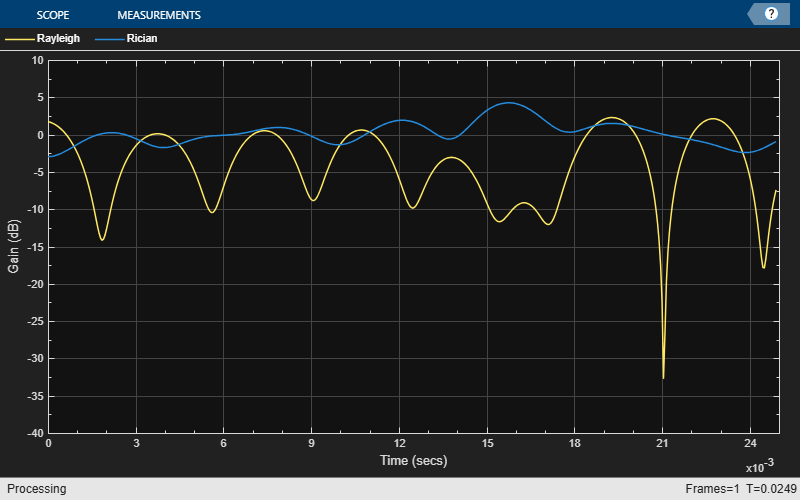### Fading Channel Impact on Signal Constellation

We now return to our original four-path Rayleigh fading channel. We use a `comm.ConstellationDiagram` System object to show the impact of narrowband fading on the signal constellation. To slow down the channel dynamics for visualization purposes, we reduce the maximum Doppler shift to 5 Hz. Compared with the QPSK channel input signal, you can observe signal attenuation and rotation at the channel output, as well as some signal distortion due to the small amount of ISI in the received signal.

```clear hRicChan hMultipathGain; release(rayChan); rayChan.PathDelays = delayVector; rayChan.AveragePathGains = gainVector; rayChan.MaximumDopplerShift = 5; % Configure a Constellation Diagram System object to show received signal constDiag = comm.ConstellationDiagram( ... 'Name', 'Received Signal After Rayleigh Fading'); numFrames = 16; for n = 1:numFrames msg = randi([0 1],bitsPerFrame,1); modSignal = qpskMod(msg); rayChanOut = rayChan(modSignal); % Display constellation diagram for Rayleigh channel output constDiag(rayChanOut); end ```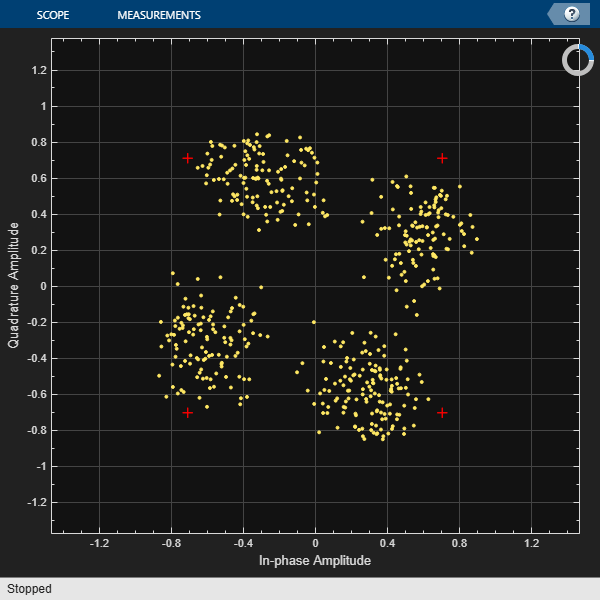When we increase the signal bandwidth to 500 kb/s (250 ksym/s), we see much greater distortion in the signal constellation. This distortion is the ISI that comes from time dispersion of the wideband signal. The channel's delay span (15 microseconds) is now larger than the QPSK symbol period (4 microseconds), so the resultant bandlimited impulse response is no longer approximately flat.

```release(rayChan); release(constDiag); rayChan.SampleRate = sampleRate500kHz; for n = 1:numFrames msg = randi([0 1],bitsPerFrame,1); modSignal = qpskMod(msg); rayChanOut = rayChan(modSignal); constDiag(rayChanOut); end ```